# 基本寻路算法

## A* 寻路算法

A*寻路是游戏程序中最常用的寻路算法，没有之一，其大致思路就是Dijkstra算法结合贪心思想。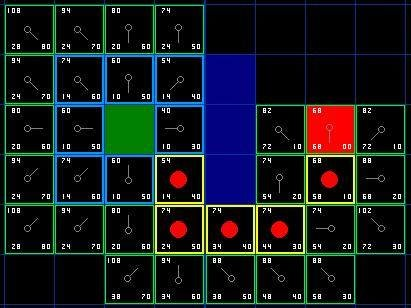A*寻路算法 - KillerAery - 博客园

## B* 寻路算法

B*算法类似于水往低处流的思路：

1. 直接往目标点移动，若遇到障碍则尝试绕爬。
2. 绕开后重复上述步骤。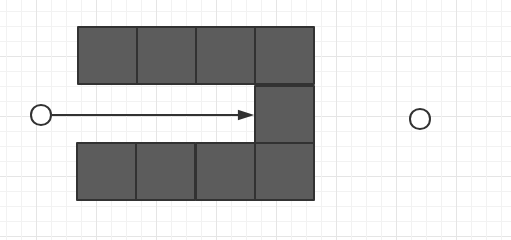## JPS/JPS+寻路算法（Jump Point Search/Plus）

JPS（jump point search）算法实际上是对A* 寻路算法的一个改进，因此在阅读本文之前需要先了解A*算法。

A* 算法在扩展节点时会把节点所有邻居都考虑进去，这样openlist中点的数量会很多，搜索效率较慢。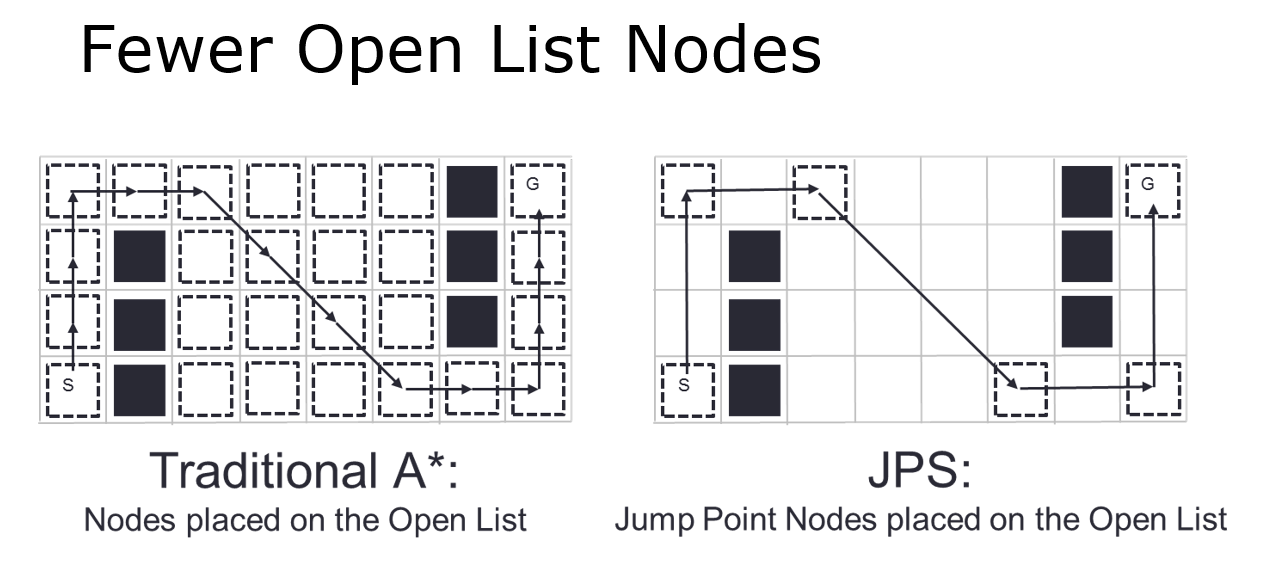JPS 算法搜到的节点总是“跳跃性”的，都是从需要改变行走方向的拐点直接跳到另一个拐点，因此这也是 Jump Point 命名的来历。

JPS+ 算法则是在JPS算法基础上多了预处理的步骤，从而使寻路更加快速。

JPS/JPS+ 寻路算法 - KillerAery - 博客园

TODO：待补充

# 寻路节点

## 使用路径点（Way Point）作为节点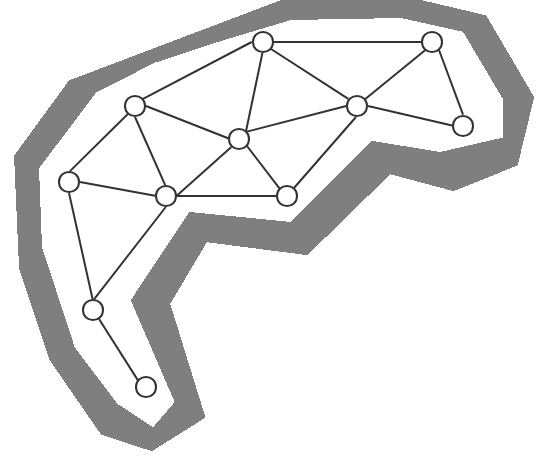（如图，使用了路径点作为节点，路径点之间的连线表示两点之间可直接移动穿过）

• 减少大量节点数量，顺带也就减少了寻路的运算速度开销。
• 相比网格节点，路径点的路径更加平滑。

## 多层次路径点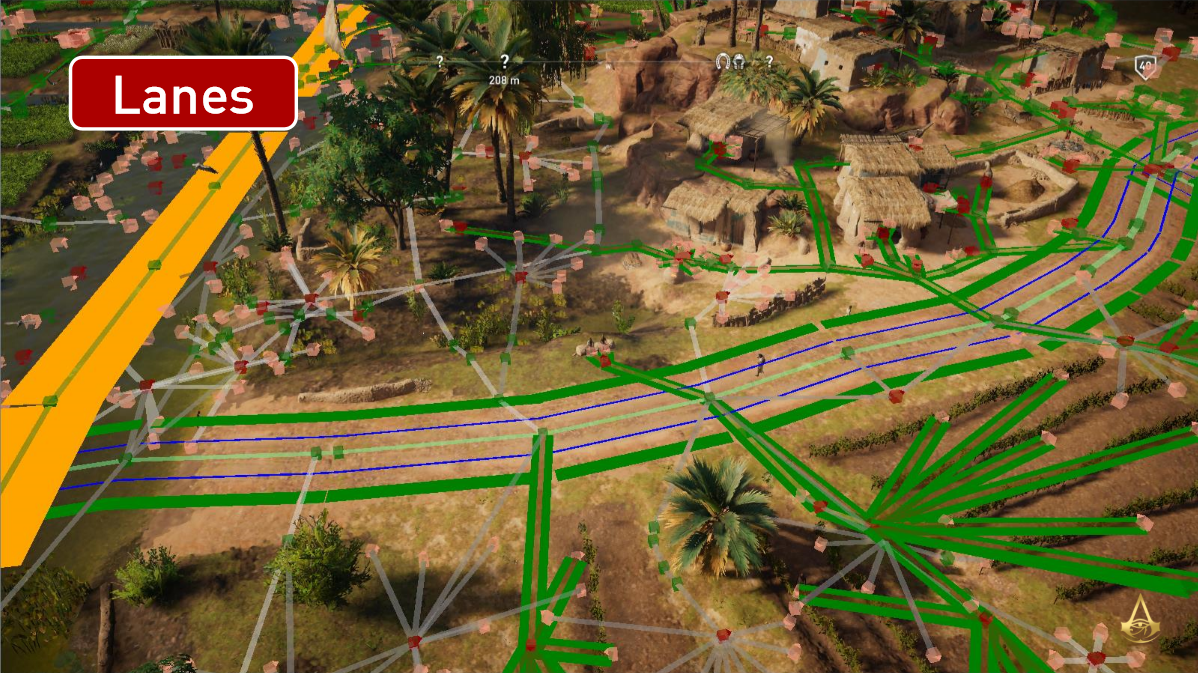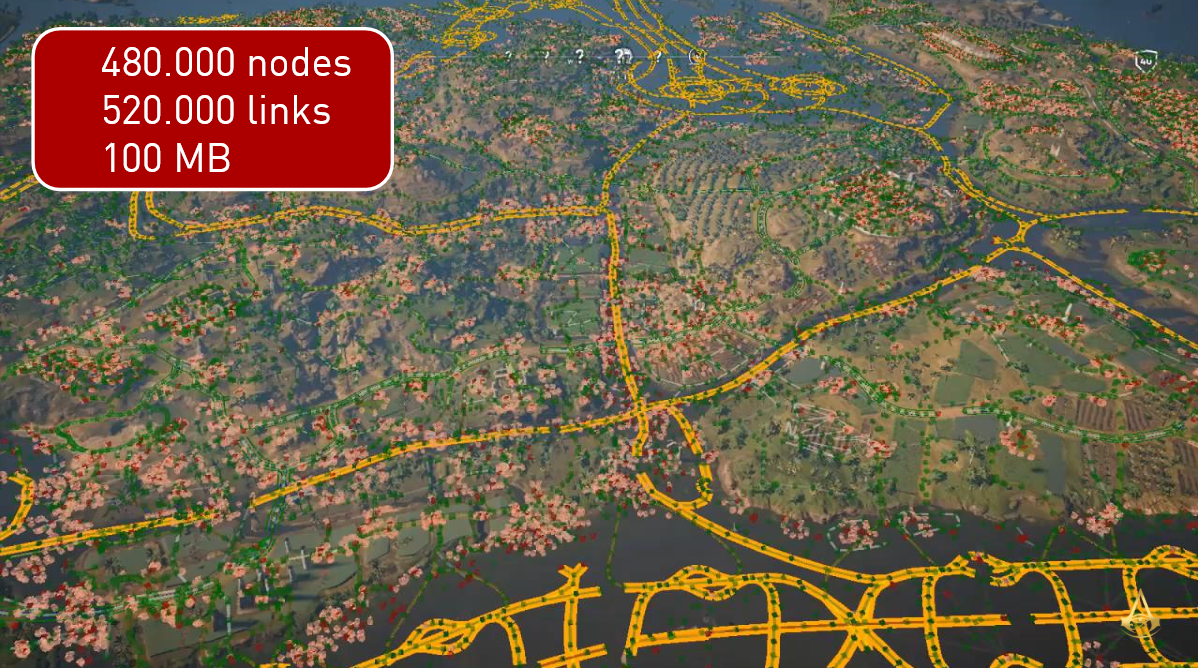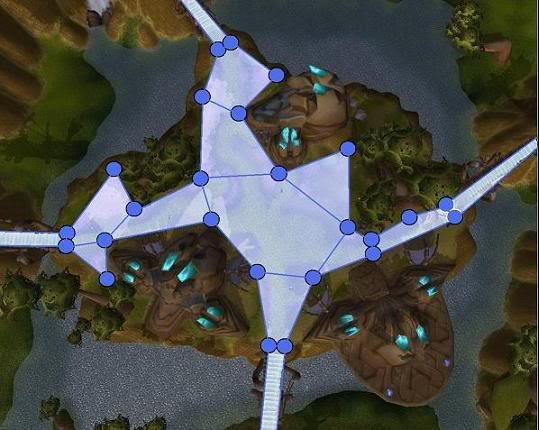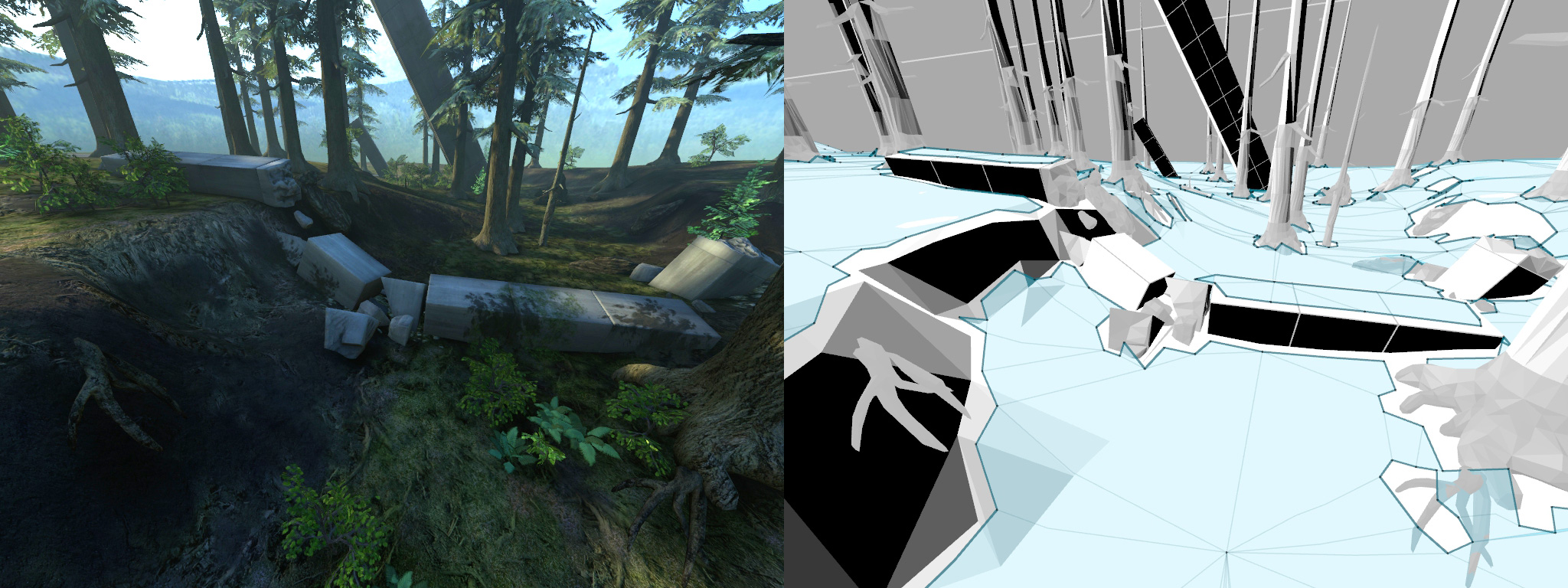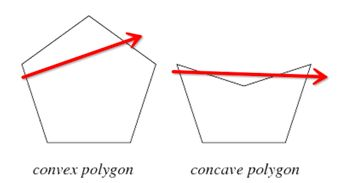• 手工划分导航网格往往工作量巨大。
• 程序化生成导航网格则实现稍微复杂。

# 预计算

## 洪水填充法（Floodfill）自动创建路径点

1.以任意一点为起始点，往周围八个方向扩展点（不能通行的位置则不扩展）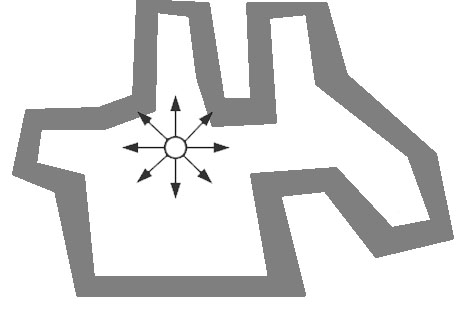2.已经扩展的点（在图中被标记成红色）不需要再次扩展，而扩展出来新的点继续扩展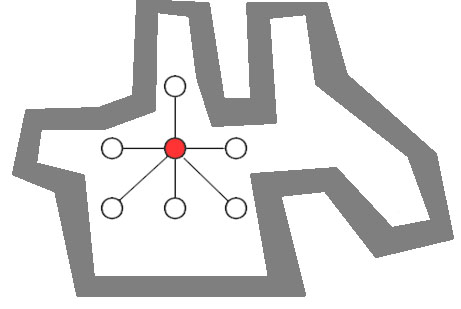3.直到所有的点都被扩展过，此时能得到一张导航图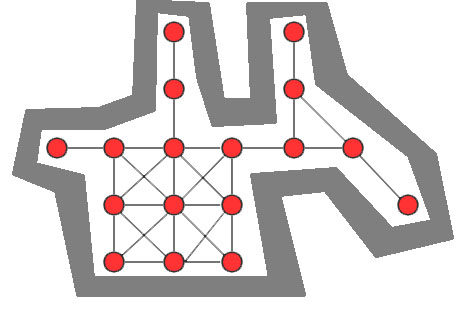//洪水填充法:从一个点开始自动生成导航图
void generateWayPoints(int beginx, int beginy, std::vector<WayPoint>& points) {
//需要探索的点的列表
std::queue<WayPoint*> pointsToExplore;
//生成起点，若受阻，不能生成路径点，则退出
if (!canGeneratePointIn(beginx, beginy))return;
points.emplace_back(WayPoint(beginx, beginy));
//扩展距离
float distance = 2.3f;
//预先写好8个方向的增量
int direction = { {1,0}, {0,1}, {0,-1}, {-1,0}, {1,1}, {-1,1}, {-1,-1},{1,-1} };
//以起点开始探索
WayPoint* begin = &points.back();
pointsToExplore.emplace(begin);
//重复探索直到探索点列表为空
while (!pointsToExplore.empty()) {
//先取出一个点开始进行探索
WayPoint* point = pointsToExplore.front();
pointsToExplore.pop();
//往8个方向探索
for (int i = 0; i < 8; ++i) {
//若当前点的目标方向连着点，则无需往这方向扩展
if (point->pointInDirection[i] == nullptr) {
continue;
}
auto x = point->x + direction[i] * distance;
auto y = point->y + direction[i] * distance;
//如果目标位置受阻，则无需往这方向扩展
if (!canGeneratePointIn(x, y)) {
continue;
}
points.emplace_back(WayPoint(x, y));
auto newPoint = &points.back();
pointsToExplore.emplace(newPoint);
//如果当前点能够无障碍通向目标点，则连接当前点和目标点
if (canWalkTo(point, newPoint)) {
point.connectToPoint(newPoint);
}
}
}
}


## 路径查询表/路径成本查询表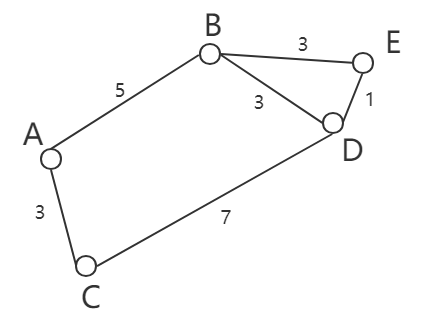（|v|为顶点数量）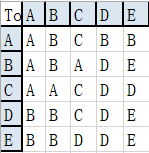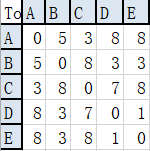## 扩展障碍碰撞几何体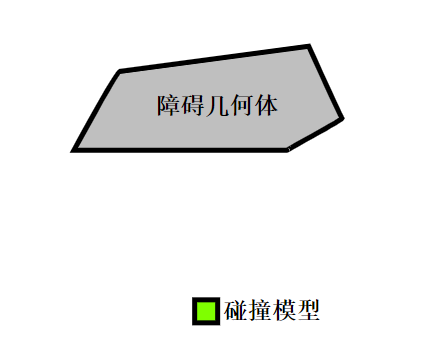• 碰撞模型的各个顶点与障碍几何体顶点重合，然后扫过去锚点形成的边界即是扩展的边界（实际上就是让碰撞模型紧挨着障碍几何体走一圈）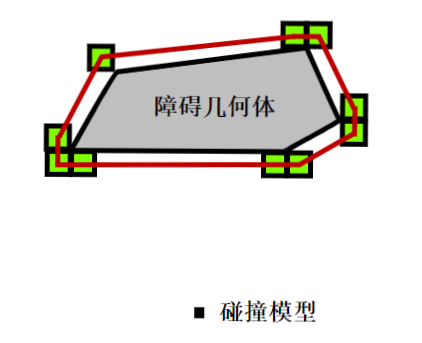• 碰撞模型的锚点与障碍几何体顶点重合，然后扫过去最外围顶点形成的边界即是扩展的边界（实际上就是让碰撞模型沿着原几何体边界走一圈）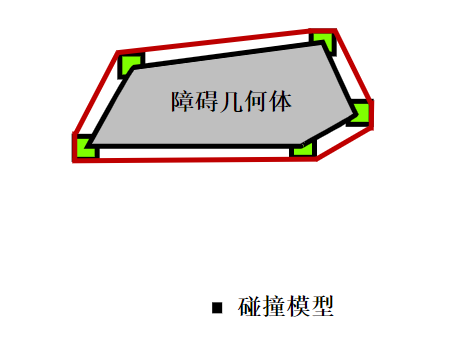• 各个需要寻路的碰撞模型最好统一形状，这样我们只需要记录一张（或少量）扩展过的障碍图。
• 碰撞模型不可以是圆形，因为这样扩展出的障碍几何体将是圆曲的，很难计算。一个解决方案是用正方形近似替代圆形来生成扩展障碍几何体。
• 当遇到非凸多边形障碍时，在凹处可能会出现扩展出的顶点重复（交点），简单的处理是凹角处不插入新的点。

## Goal Bounding

Goal Bounding是一种节点裁剪技术，可加快寻路速度。Goal Bounding为每个节点的边（Edge）计算一个节点集合，该集合至少包含通过该边可到达最短路的所有节点。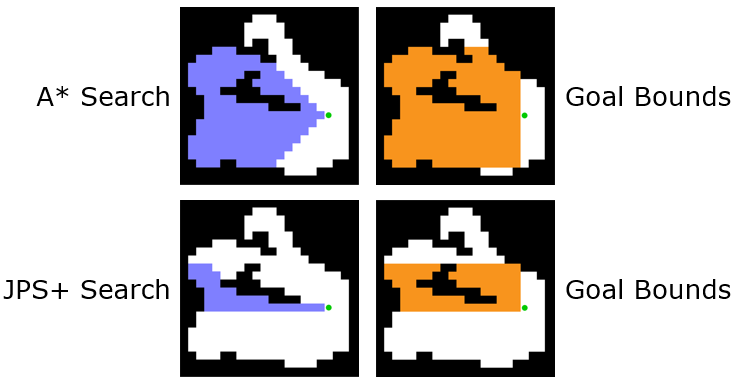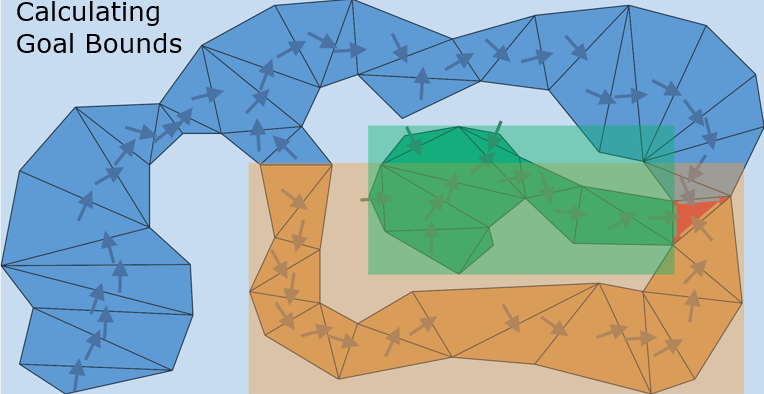• 在通过某个边缘（即朝某个方向）搜索时，只有目标节点包含在通过该边的节点集合时，才会搜索该方向，否则该方向没必要搜索。
• Goal Bounding 可以使用在任何寻路算法（Dijkstra、A*、JPS+等）应用于任何搜索空间（网格，图形，NavMesh等）。
• Goal Bounding 的缺点在于必须使用$$O(n^2)$$时间对 Goal Bounding 数据进行预处理，因此无法支持对搜索空间进行动态运行时修改（添加或删除边/墙）。其次，Goal Bounding 要求$$O(n)$$的存储空间（Goal Bounds形状为AABB盒且地图为网格时，每个节点有8个边，每个边需要存储4个值，即总共 $$32n$$ 个值）。

1. 从该节点出发，先朝八个方向各移动一格，将8个方向移动后的位置记录在对应的8个队列里。（这一步是为了保证搜索通过了目标方向。）
2. 8个队列进行一轮移动：每个队列取出队首节点后，从该节点向8个方向移动一格。每移动到新的位置则记录在集合里，重复走过的位置（若是本轮中其它队列走过的位置，则依然视为本队列的新位置）则不需再记录。此外，每个队列每个取出过的节点需放入对应的集合（8个集合）。
3. 重复步骤2，直到八个队列都为空，这意味着无论哪个方向都走到了尽头（边界或障碍）。
4. 对其中一个集合进行遍历，提取出其中最大、最小的y值和最大、最小的x值，这个便作为Goal Bounds的AABB形状的表示。此时便代表本节点的一个方向的Goal Bounds计算完毕。
5. 重复步骤4，直到本节点八个方向的Goal Bounds都计算完毕。

TODO 待更新

# 启发函数

## A*算法是最优解吗？

$$f_2(n_{end}) = c_2$$

$$f_1(n) <= c_1 < c_2 = f_2(n_{end})$$

• $$h(n) = 0$$，意味着 $$f(n) = g(n)$$，此时A星算法则退化成了Dijkstra算法，效率十分低。
• $$h(n) < cost(n)$$，搜索效率略低，h(n)越小，意味着搜索节点越多，效率上越低。
• $$h(n) = cost(n)$$，是A*算法最高效的情形。
• $$h(n) > cost(n)$$，不可以保证一致性，$$h(n)$$ 越小，寻得的路越接近最优解。

• 如果只允许朝上下左右四个方向移动，则使用曼哈顿距离（Manhattan distance）。
• 如果允许朝任何方向（或八个方向）移动，则使用欧几里得距离（Euclidean distance）。

## 战术评估

• 地形优势：例如平地节点走得更快而山地节点走得更慢。
• 视野优势：某些地方具有良好的视野（例如高地），AI需要准备战斗时应该倾向占领视野优势点。
• 战术优势：一些地方（例如刷出医疗包的地点，可操控机枪）提供了战术优势，AI应倾向占领这些战术优势地点。
• 其他...

## 加权优化

A*算法按照传统的启发函数进行路径搜索时，会不断往返搜索，搜索节点过多。如果我们减轻离终点比较接近的节点启发函数值，那么A*算法会更有可能优先开启这些节点，这就是加权优化的核心思想。

$$f(n)=g(n)+k*h(n)$$

$$f(n)=g(n)+ exp⁡[h(n)]*h(n)$$

• 当h(n)较大时，权重大，这样使节点迅速向终点行进。
• 当h(n)较小时，权重小。
• 终点附近时，权重接近1，可保证终点可达。

1. 当节点离终点较远时，权重应该大一些；当节点逐渐靠近终点时，权重随之变小。
2. 尽可能让启发函数值不要太大（小于等于实际路径代价是最好的，可有一致性）。
3. 当节点到达终点时，启发函数值 $$f(n)$$ 等于实际值。

# 其它改进

## 平均帧运算

（虽然有所缓慢，但是就几帧的东西，一般实际玩家的体验不会有大影响）

## 路径平滑

（下图为一次基于网格的正常寻路算法结果得到的路径）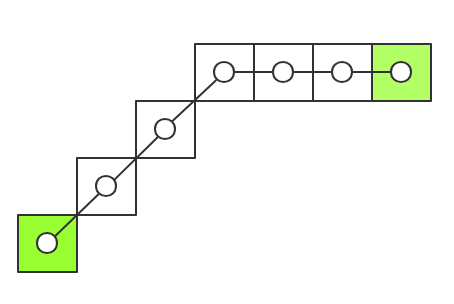（下图为理想中的平滑路径）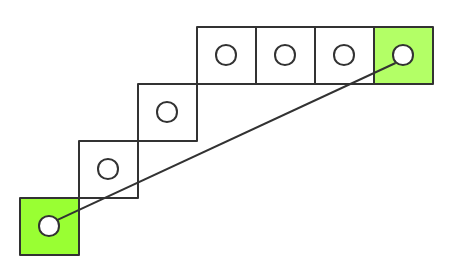• 快速而粗糙的平滑

void fastSmooth(std::list<OpenPoint*>& path) {
//先获取p1,p2,p3，分别代表顺序的第一/二/三个迭代元素。
auto p1 = path.begin();
auto p2 = p1; ++p2;
auto p3 = p2; ++p2;
while (p3 != path.end()) {
//若p1能直接走到p3，则移除p2，并将p2,p3往后一位
// aa-bb-cc-dd-...  =>  aa-cc-dd-...
// p1 p2 p3             p1 p2 p3
if (CanWalkBetween(p1, p3)) {
++p3;
p2 = path.erase(p2);
}
//若不能走到，则将p1,p2,p3都往后一位。
// aa-bb-cc-dd-...  =>  aa-bb-cc-dd-...
// p1 p2 p3                p1 p2 p3
else {
++p1;
++p2;
++p3;
}
}
}

• 精准而慢的平滑

void preciseSmooth(std::list<OpenPoint*>& path) {
auto p1 = path.begin();
while (p1 != path.end()) {
auto p3 = p1; ++p3; ++p3;
while (p3 != path.end()) {
//若p1能直接走到p3，则移除p1和p3之间的所有点,并将p3往后一位
if (CanWalkBetween(p1, p3)) {
auto deleteItr = p1; ++deleteItr;
p3 = path.erase(deleteItr,p3);
}
//否则，p3往后一位
else {
++p3;
}
}
//推进一位
++p1;
}
}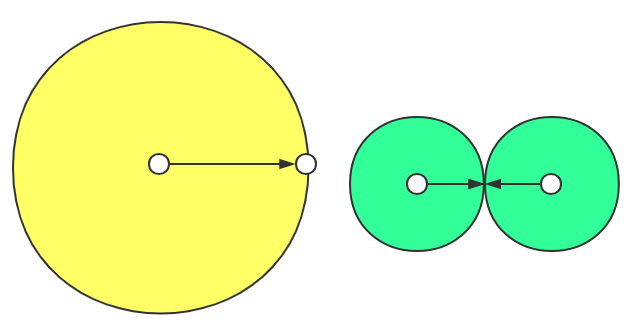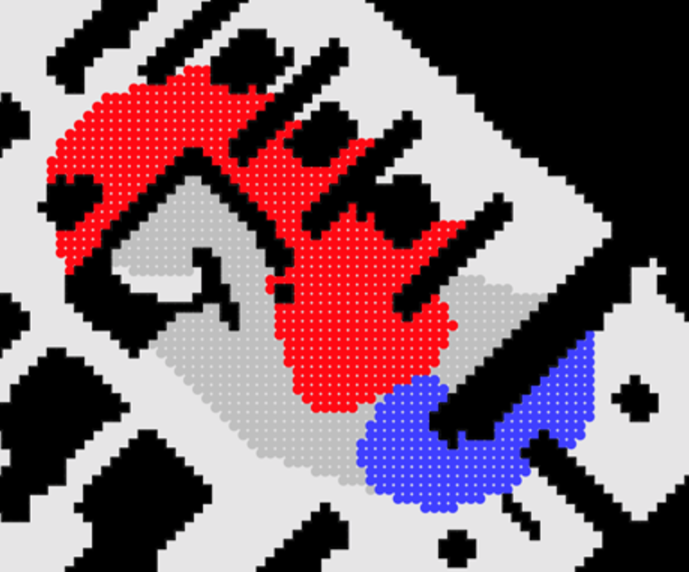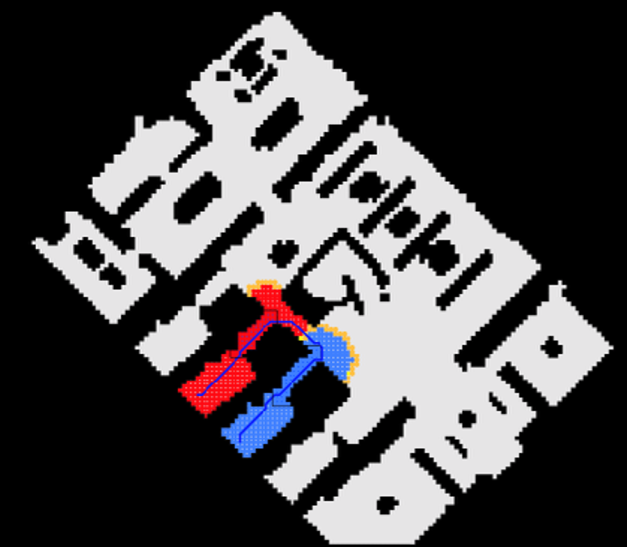## 路径拼接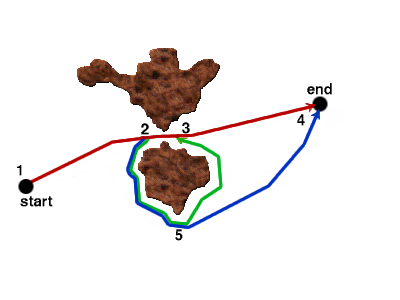M不影响CPU时间，而影响了响应时间和路径质量的折衷：

• 如果M太大，物体的移动将不能快速对地图的改变作出反应。
• 如果M太小，拼接的路径可能太短以致于不能正确地绕过障碍物，出现不理想的路径，如（1 -> 2 -> 5 -> 3 -> 4）。

• 若经常发现这种情况出现，那么重新计算整条路径也不失为一个解决办法。
• 尝试使用不同的M值和不同的拼接频率K(如每 $$\frac{3}{4}M$$ 步)以用于不同的情形。
• 此外应该使用栈来反向保存路径，因为删除和拼接都是在路径尾部进行的。

# 参考

posted @ 2019-02-01 21:12  KillerAery  阅读(8555)  评论(0编辑  收藏  举报